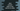# How to convert miles to kilometers and kilometers to miles in Java## How to convert miles to kilometers and kilometers to miles in Java:

In this Java progarm, we will learn how to convert miles to kilometers and kilometers to miles using user given values. The program will ask the user to enter the value, it will convert that and print that result back.

### Algorithm to use:

It is easy. 1 mile is equal to 1.60934 kilometers. So, if we are converting a mile value to kilometer, we can multiply that value by 1.60934 to get the result. Or, if we are converting a kilometer to miles, we need to divide the value by 1.60934.

## Java program:

This program can handle both miles and kilometer inputs. It will ask the user to enter the input type and based on the input type, it will ask the user to enter miles value or kilometer value.

``````import java.util.Scanner;

public class Main {

public static void main(String[] args) {
char type;
double m, km;

Scanner sc = new Scanner(System.in);

System.out.println("Enter 'm' to convert miles to kilometer and 'k' to convert kilometer to miles: ");
type = sc.nextLine().charAt(0);

if (type == 'm') {
System.out.println("Enter the miles value: ");
m = sc.nextDouble();
km = 1.60934 * m;

System.out.println(m + " miles = " + km + " kilometers");
} else if (type == 'k') {
System.out.println("Enter the kilometers value: ");
km = sc.nextDouble();
m = km / 1.60934;

System.out.println(km + " kilometers = " + m + " miles");
} else {
System.out.println("Please enter a valid input !");
}
}
}``````

Here,

• type is a character variable to hold the type of conversion.
• m and km are two double variables to hold the meter and kilometer values.
• sc is a Scanner object to read the user input.
• It asks the user to enter m to do miles to kilometer conversion and k to do kilometer to miles conversion.
• If the user enter m, it executes the code inside the if block, it executes the code inside the else if block if the user enters k and else it moves to the else block.
• It asks the user to enter the miles or kilometers, converts it and print both values back.

### Sample output:

If you run this program, it will print output as like below:

``````Enter 'm' to convert miles to kilometer and 'k' to convert kilometer to miles:
k
Enter the kilometers value:
40.2335
40.2335 kilometers = 25.0 miles

Enter 'm' to convert miles to kilometer and 'k' to convert kilometer to miles:
m
Enter the miles value:
25
25.0 miles = 40.2335 kilometers``````

## Method 2: By using different methods:

We can create different methods to do the conversion, i.e. one method for miles to kilometer and another for kilometer to miles.

``````package com.company;

import java.util.Scanner;

public class Main {

public static double milesToKm(double miles) {
return 1.60934 * miles;
}

public static double kmToMiles(double km) {
return km / 1.60934;
}

public static void main(String[] args) {
char type;
double m, km;

Scanner sc = new Scanner(System.in);

System.out.println("Enter 'm' to convert miles to kilometer and 'k' to convert kilometer to miles: ");
type = sc.nextLine().charAt(0);

if (type == 'm') {
System.out.println("Enter the miles value: ");
m = sc.nextDouble();
km = milesToKm(m);

System.out.println(m + " miles = " + km + " kilometers");
} else if (type == 'k') {
System.out.println("Enter the kilometers value: ");
km = sc.nextDouble();
m = kmToMiles(km);

System.out.println(km + " kilometers = " + m + " miles");
} else {
System.out.println("Please enter a valid input !");
}
}
}``````
• milesToKm method takes the miles value as its parameter, converts it to kilometers and returns that value.
• kmToMiles method takes the kilometer value as its parameter, converts it to miles and returns that value.
• We are calling these methods instead of calculating the conversion directly.

If you run the above program, it will give similar result.